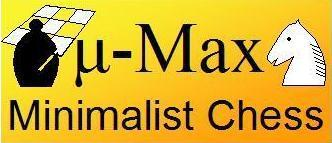```Previous   Next
```

## Search: Do and Undo Move

#### Doing a Move

The move generation delivers the start and target squares x and y, and the capture square H. Performing the move is simply clearing the capture square, putting the moving piece (u) in the target square, and clearing the start square. In that order, since the capture square and target square usually coincide. The virgin bit is stripped off the piece code, to indicate that the piece has moved. If the move was a castling, we have to move the Rook as well, (from G to F), since start and target square only give the move of the King in that case.

#### And Taking it Back

Undoing the move is just as easy: Clear the target square and put the captured piece t back (in that order!), and put the moving piece back on its start square. Because the moving piece is remembered in the variable u, we don't have to read it from the board, and automatically restore the virgin bit to its state from before the move (and turn a promotion Queen back into a Pawn). In this case, we always move a Rook back as well, from F to G. We can afford that, because for non-castlings G is the dummy square, and we don't care if there is a Rook there. The square F that is cleared by this Rook move is usually also the dummy square. Only in the case of an e.p. capture it contains a valid square number, but then that square was empty already, and clearing it changes nothing.

Below the code that performs the moves and takes them back is highlighted:

```/***************************************************************************/
/*                               micro-Max,                                */
/* A chess program smaller than 2KB (of non-blank source), by H.G. Muller  */
/***************************************************************************/
/* version 3.2 (2000 characters) features:                                 */
/* - recursive negamax search                                              */
/* - quiescence search with recaptures                                     */
/* - recapture extensions                                                  */
/* - (internal) iterative deepening                                        */
/* - best-move-first 'sorting'                                             */
/* - a hash table storing score and best move                              */
/* - full FIDE rules (expt minor ptomotion) and move-legality checking     */

#define F(I,S,N) for(I=S;I<N;I++)
#define W(A) while(A)
>#define K(A,B) *(int*)(T+A+(B&8)+S*(B&7))
#define J(A) K(y+A,b[y])-K(x+A,u)-K(H+A,t)

#define U 16777224
struct _ {int K,V;char X,Y,D;} A[U];           /* hash table, 16M+8 entries*/

int V=112,M=136,S=128,I=8e3,C=799,Q,N,i;       /* V=0x70=rank mask, M=0x88 */

char O,K,L,
w[]={0,1,1,3,-1,3,5,9},                        /* relative piece values    */
o[]={-16,-15,-17,0,1,16,0,1,16,15,17,0,14,18,31,33,0, /* step-vector lists */
7,-1,11,6,8,3,6,                          /* 1st dir. in o[] per piece*/
6,3,5,7,4,5,3,6},                         /* initial piece setup      */
b,                                        /* board: half of 16x8+dummy*/
T,                                       /* hash translation table   */

n[]=".?+nkbrq?*?NKBRQ";                        /* piece symbols on printout*/

D(k,q,l,e,J,Z,E,z,n)    /* recursive minimax search, k=moving side, n=depth*/
int k,q,l,e,J,Z,E,z,n;  /* (q,l)=window, e=current eval. score, E=e.p. sqr.*/
{                       /* e=score, z=prev.dest; J,Z=hashkeys; return score*/
int j,r,m,v,d,h,i=8,F,G;
char t,p,u,x,y,X,Y,H,B;
struct _*a=A;
/* lookup pos. in hash table*/
j=(k*E^J)&U-9;                                /* try 8 consec. locations  */
while((h=A[++j].K)&&h-Z&&--i);                /* first empty or match     */
a+=i?j:0;                                     /* dummy A if miss & full*/
if(a->K)                                      /* hit: pos. is in hash tab */
{d=a->D;v=a->V;X=a->X;                        /* examine stored data      */
if(d>=n)                                     /* if depth sufficient:     */
{if(v>=l|X&S&&v<=q|X&8)return v;             /* use if window compatible */
d=n-1;                                      /* or use as iter. start    */
}X&=~M;Y=a->Y;                               /*      with best-move hint */
Y=d?Y:0;                                     /* don't try best at d=0    */
}else d=X=Y=0;                                /* start iter., no best yet */
N++;                                          /* node count (for timing)  */
W(d++<n|z==8&N<1e7&d<98)                      /* iterative deepening loop */
{x=B=X;                                       /* start scan at prev. best */
Y|=8&Y>>4;                                   /* request try noncastl. 1st*/
m=d>1?-I:e;                                  /* unconsidered:static eval */
do{u=b[x];                                   /* scan board looking for   */
if(u&k)                                     /*  own piece (inefficient!)*/
{r=p=u&7;                                   /* p = piece type (set r>0) */
j=o[p+16];                                 /* first step vector f.piece*/
W(r=p>2&r<0?-r:-o[++j])                    /* loop over directions o[] */
{A:                                        /* resume normal after best */
y=x;F=G=S;                                /* (x,y)=move, (F,G)=castl.R*/
do{H=y+=r;                                /* y traverses ray          */
if(Y&8)H=y=Y&~M;                         /* sneak in prev. best move */
if(y&M)break;                            /* board edge hit           */
if(p<3&y==E)H=y^16;                      /* shift capt.sqr. H if e.p.*/
t=b[H];if(t&k|p<3&!(r&7)!=!t)break;      /* capt. own, bad pawn mode */
i=99*w[t&7];                             /* value of capt. piece t   */
if(i<0||E-S&&b[E]&&y-E<2&E-y<2)m=I;      /* K capt. or bad castling  */
if(m>=l)goto C;                          /* abort on fail high       */

if(h=d-(y!=z))                           /* remaining depth(-recapt.)*/
{v=p<6?b[x+8]-b[y+8]:0;                  /* center positional pts.   */
b[G]=b[H]=b[x]=0;b[y]=u&31;             /* do move, strip virgin-bit*/
if(!(G&M)){b[F]=k+6;v+=30;}             /* castling: put R & score  */
if(p<3)                                 /* pawns:                   */
{v-=9*(((x-2)&M||b[x-2]!=u)+            /* structure, undefended    */
((x+2)&M||b[x+2]!=u)-1);         /*        squares plus bias */
if(y+r+1&S){b[y]|=7;i+=C;}             /* promote p to Q, add score*/
}
v=-D(24-k,-l-(l>e),m>q?-m:-q,-e-v-i,    /* recursive eval. of reply */
J+J(0),Z+J(8)+G-S,F,y,h);          /* J,Z: hash keys           */
v-=v>e;                                 /* delayed-gain penalty     */
if(z==9)                                /* called as move-legality  */
{if(v!=-I&x==K&y==L)                    /*   checker: if move found */
{Q=-e-i;O=F;return l;}                 /*   & not in check, signal */
v=m;                                   /* (prevent fail-lows on    */
}                                       /*   K-capt. replies)       */
b[G]=k+38;b[F]=b[y]=0;b[x]=u;b[H]=t;    /* undo move,G can be dummy */
if(Y&8){m=v;Y&=~8;goto A;}              /* best=1st done,redo normal*/
if(v>m){m=v;X=x;Y=y|S&G;}               /* update max, mark with S  */
}                                        /*          if non castling */
t+=p<5;                                  /* fake capt. for nonsliding*/
if(p<3&6*k+(y&V)==S                      /* pawn on 3rd/6th, or      */
||(u&~24)==36&j==7&&                 /* virgin K moving sideways,*/
G&M&&b[G=(x|7)-(r>>1&7)]&32          /* 1st, virgin R in corner G*/
&&!(b[G^1]|b[G^2])                   /* 2 empty sqrs. next to R  */
){F=y;t--;}                              /* unfake capt., enable e.p.*/
}W(!t);                                   /* if not capt. continue ray*/
}}}W((x=x+9&~M)-B);                          /* next sqr. of board, wrap */
C:if(m>I/4|m<-I/4)d=99;                        /* mate is indep. of depth  */
m=m+I?m:-D(24-k,-I,I,0,J,K,S,z,1)/2;         /* best loses K: (stale)mate*/
if(!a->K|(a->X&M)!=M|a->D<=d)                /* if new/better type/depth:*/
{a->K=Z;a->V=m;a->D=d;A->K=0;                /* store in hash,dummy stays*/
a->X=X|8*(m>q)|S*(m<l);a->Y=Y;              /* empty, type (limit/exact)*/
}                                            /*    encoded in X S,8 bits */
/*if(z==8)printf("%2d ply, %9d searched, %6d by (%2x,%2x)\n",d-1,N,m,X,Y&0x77);*/
}
if(z&8){K=X;L=Y&~M;}
return m;
}

main()
{
int j,k=8,*p,c;

F(i,0,8)
{b[i]=(b[i+V]=o[i+24]+40)+8;b[i+16]=18;b[i+96]=9;   /* initial board setup*/
F(j,0,8)b[16*j+i+8]=(i-4)*(i-4)+(j-3.5)*(j-3.5);   /* center-pts table   */
}                                                   /*(in unused half b[])*/
F(i,M,1035)T[i]=random()>>9;

W(1)                                                /* play loop          */
{F(i,0,121)printf(" %c",i&8&&(i+=7)?10:n[b[i]&15]); /* print board        */
p=c;W((*p++=getchar())>10);                        /* read input line    */
N=0;
if(*c-10){K=c-16*c+C;L=c-16*c+C;}else  /* parse entered move */
D(k,-I,I,Q,1,1,O,8,0);                            /* or think up one    */
F(i,0,U)A[i].K=0;                                  /* clear hash table   */
if(D(k,-I,I,Q,1,1,O,9,2)==I)k^=24;                 /* check legality & do*/
}
}
```
```Previous   Next
```